# Chapter 17 THERMODYNAMICS What is Thermodynamics Thermodynamics is

• Slides: 28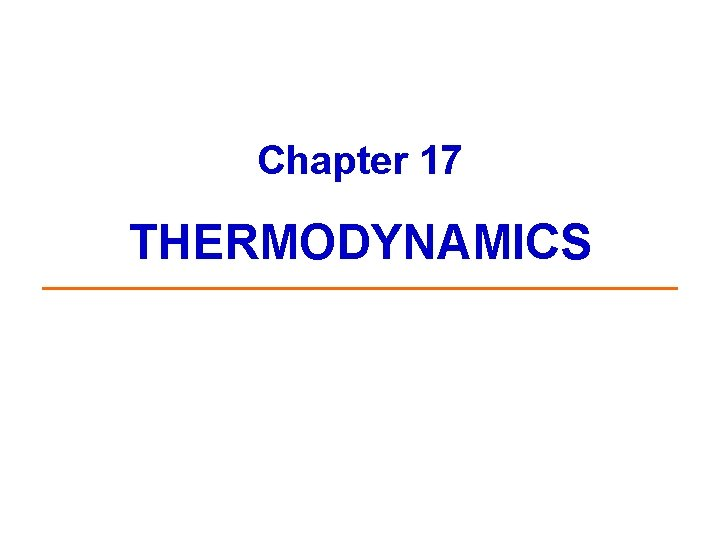Chapter 17 THERMODYNAMICSWhat is Thermodynamics? Thermodynamics is the study of energy changes that accompany physical and chemical processes. Word origin: “Thermo”, from temperature, meaning heat “Dynamics”, which means motion (under the action of forces) Chemical thermodynamics answers the questions: v How much heat is evolved during a chemical reaction ("thermochemistry")? v What determines the direction of spontaneous chemical reactions?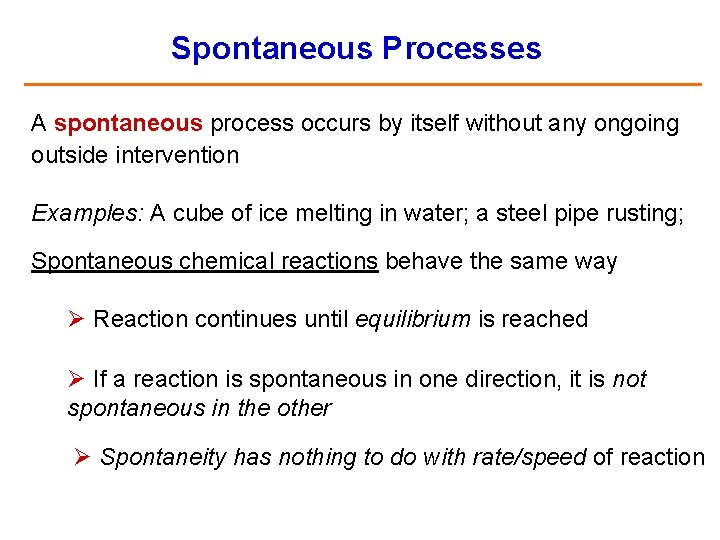Spontaneous Processes A spontaneous process occurs by itself without any ongoing outside intervention Examples: A cube of ice melting in water; a steel pipe rusting; Spontaneous chemical reactions behave the same way Ø Reaction continues until equilibrium is reached Ø If a reaction is spontaneous in one direction, it is not spontaneous in the other Ø Spontaneity has nothing to do with rate/speed of reaction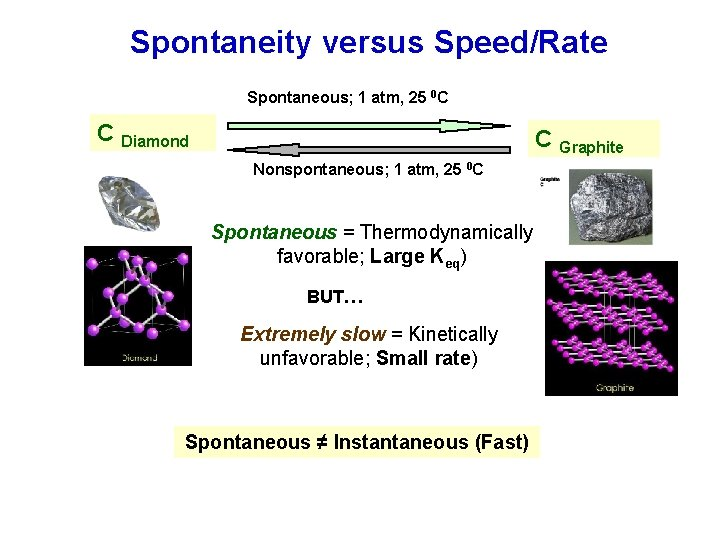Spontaneity versus Speed/Rate Spontaneous; 1 atm, 25 0 C C Diamond C Graphite Nonspontaneous; 1 atm, 25 0 C Spontaneous = Thermodynamically favorable; Large Keq) BUT… Extremely slow = Kinetically unfavorable; Small rate) Spontaneous ≠ Instantaneous (Fast)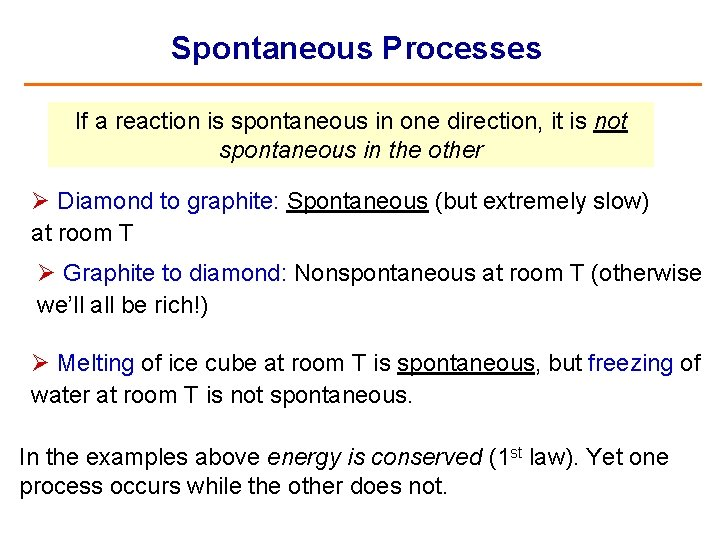Spontaneous Processes If a reaction is spontaneous in one direction, it is not spontaneous in the other Ø Diamond to graphite: Spontaneous (but extremely slow) at room T Ø Graphite to diamond: Nonspontaneous at room T (otherwise we’ll all be rich!) Ø Melting of ice cube at room T is spontaneous, but freezing of water at room T is not spontaneous. In the examples above energy is conserved (1 st law). Yet one process occurs while the other does not.Spontaneous Processes (Cont. ) Spontaneous processes may need a little “push” to get started Example: Hydrogen and oxygen gases burn spontaneously only after being ignited by a spark 2 H 2 (g) + O 2 (g) 2 H 2 O(l) Ø The reverse reaction is nonspontaneous (i. e. Water does not simply decompose into H 2 and O 2 gases)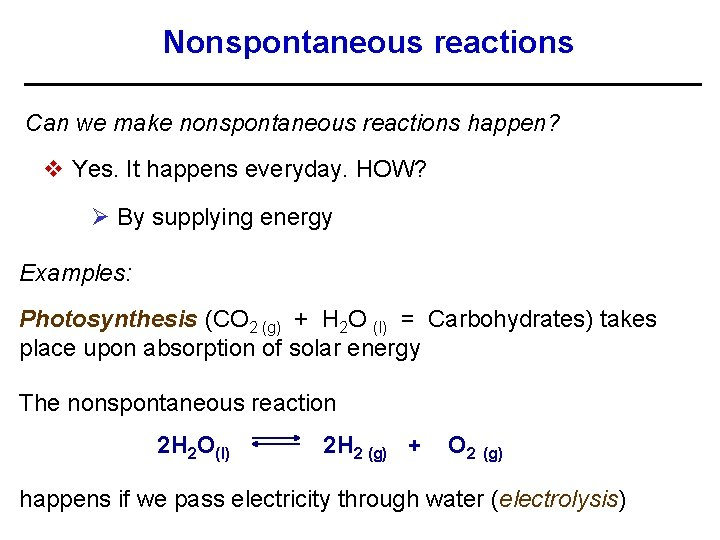Nonspontaneous reactions Can we make nonspontaneous reactions happen? v Yes. It happens everyday. HOW? Ø By supplying energy Examples: Photosynthesis (CO 2 (g) + H 2 O (l) = Carbohydrates) takes place upon absorption of solar energy The nonspontaneous reaction 2 H 2 O(l) 2 H 2 (g) + O 2 (g) happens if we pass electricity through water (electrolysis)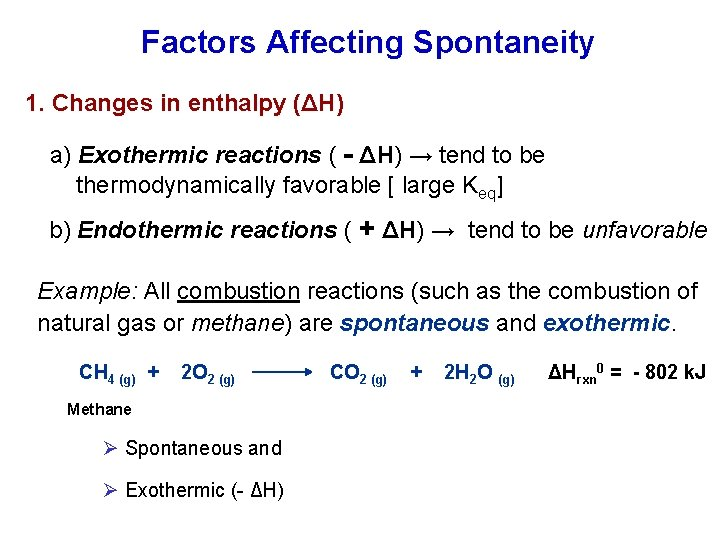Factors Affecting Spontaneity 1. Changes in enthalpy (ΔH) a) Exothermic reactions ( - ΔH) → tend to be thermodynamically favorable [ large Keq] b) Endothermic reactions ( + ΔH) → tend to be unfavorable Example: All combustion reactions (such as the combustion of natural gas or methane) are spontaneous and exothermic. CH 4 (g) + 2 O 2 (g) CO 2 (g) + 2 H 2 O (g) ΔHrxn 0 = - 802 k. J Methane Ø Spontaneous and Ø Exothermic (- ΔH)Factors Affecting Spontaneity – Cont. NOTE: Not all exothermic reactions are spontaneous Examples of endothermic reactions that are spontaneous Ø Vaporization of water (+ΔH) at ordinary T and P Ø Dissolving of Na. Cl in water (+ΔH) Thus, the sign of ΔH does not always predict spontaneous change Q. What other factor affects direction of spontaneous reactions?Factors Affecting Spontaneity – Cont. 2. Changes in entropy (ΔS) or degree of “disorder” Recall: Entropy (S) is a measure of the dispersal of energy as a function of temperature Ø Closely associated with randomness or disorder a) Increase in entropy ( + ΔS) or disorder → tend to be favorable [ large Keq] b) Decrease in entropy ( - ΔS) or more order → tend to be unfavorable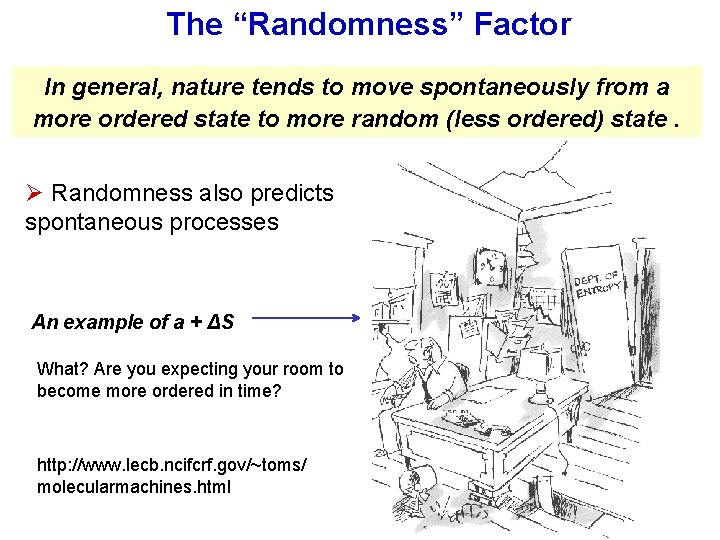The “Randomness” Factor In general, nature tends to move spontaneously from a more ordered state to more random (less ordered) state. Ø Randomness also predicts spontaneous processes An example of a + ΔS What? Are you expecting your room to become more ordered in time? http: //www. lecb. ncifcrf. gov/~toms/ molecularmachines. htmlFactors Affecting Spontaneity – Cont. Change to more disordered (more random) state = Increase in entropy (+ ΔS) Q. Which of the two images below has a + ΔS? Is spontaneous above 0 0 C? Image source: http: //demo. physics. uiuc. edu/Lect Demo/scripts/demo_descript. idc? Demo. ID=1114 MELTING v More disorder = higher entropy (+ ΔS) v Spontaneous when T > 0 0 C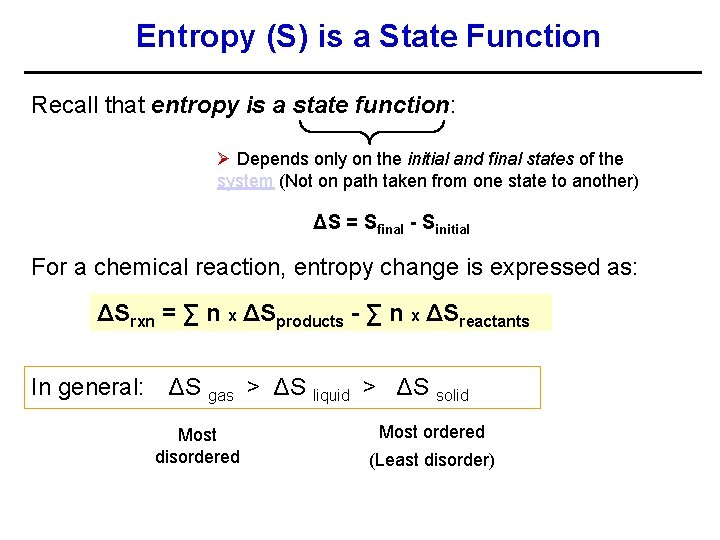Entropy (S) is a State Function Recall that entropy is a state function: Ø Depends only on the initial and final states of the system (Not on path taken from one state to another) ΔS = Sfinal - Sinitial For a chemical reaction, entropy change is expressed as: ΔSrxn = ∑ n x ΔSproducts - ∑ n x ΔSreactants In general: ΔS gas > ΔS liquid > ΔS solid Most disordered Most ordered (Least disorder)Entropy (S) and Phase Changes Exercise: Predict the sign of ΔS in each of the following processes. Freezing - ΔS Vaporization + ΔS (becomes more disordered) Condensation - ΔS Reactions that produce gas (+ ΔS) tend to be favorable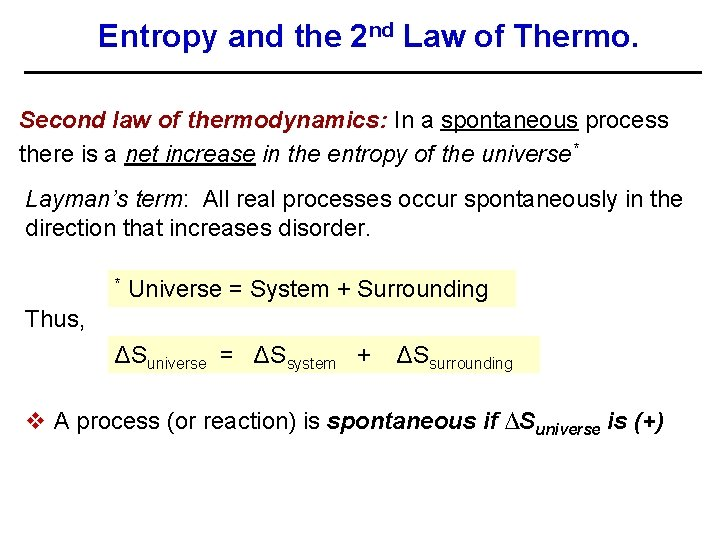Entropy and the 2 nd Law of Thermo. Second law of thermodynamics: In a spontaneous process there is a net increase in the entropy of the universe* Layman’s term: All real processes occur spontaneously in the direction that increases disorder. * Universe = System + Surrounding Thus, ΔSuniverse = ΔSsystem + ΔSsurrounding v A process (or reaction) is spontaneous if ∆Suniverse is (+)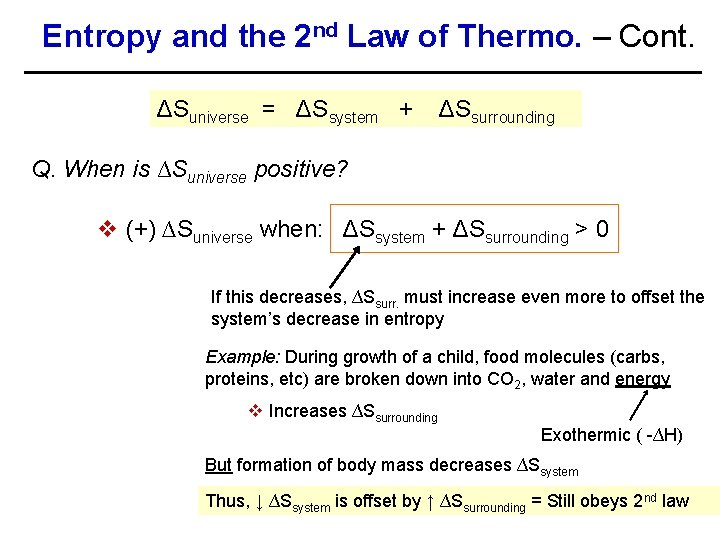Entropy and the 2 nd Law of Thermo. – Cont. ΔSuniverse = ΔSsystem + ΔSsurrounding Q. When is ∆Suniverse positive? v (+) ∆Suniverse when: ΔSsystem + ΔSsurrounding > 0 If this decreases, ∆Ssurr. must increase even more to offset the system’s decrease in entropy Example: During growth of a child, food molecules (carbs, proteins, etc) are broken down into CO 2, water and energy v Increases ∆Ssurrounding Exothermic ( -∆H) But formation of body mass decreases ∆Ssystem Thus, ↓ ∆Ssystem is offset by ↑ ∆Ssurrounding = Still obeys 2 nd law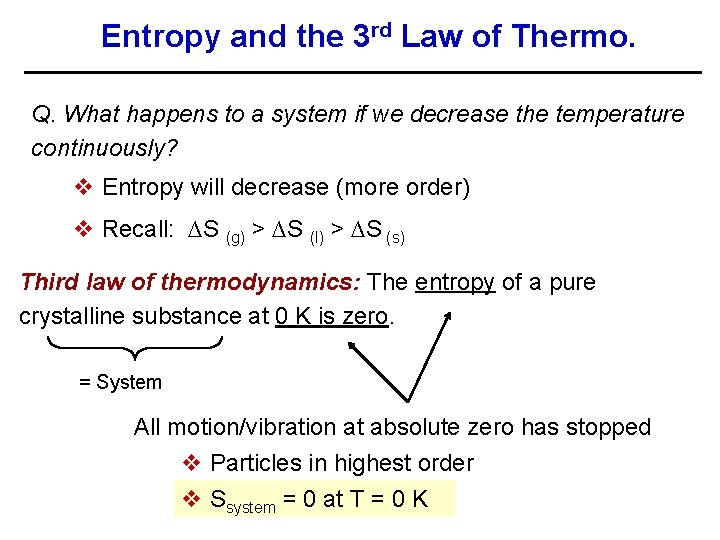Entropy and the 3 rd Law of Thermo. Q. What happens to a system if we decrease the temperature continuously? v Entropy will decrease (more order) v Recall: ∆S (g) > ∆S (l) > ∆S (s) Third law of thermodynamics: The entropy of a pure crystalline substance at 0 K is zero. = System All motion/vibration at absolute zero has stopped v Particles in highest order v Ssystem = 0 at T = 0 K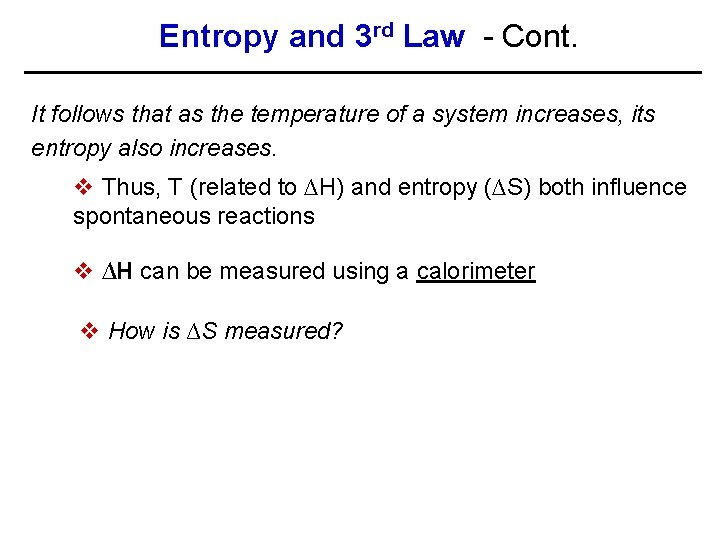Entropy and 3 rd Law - Cont. It follows that as the temperature of a system increases, its entropy also increases. v Thus, T (related to ∆H) and entropy (∆S) both influence spontaneous reactions v ∆H can be measured using a calorimeter v How is ∆S measured?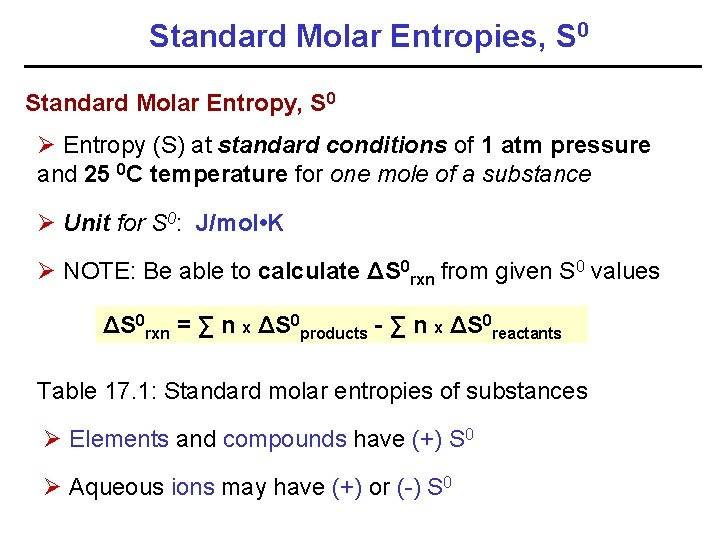Standard Molar Entropies, S 0 Standard Molar Entropy, S 0 Ø Entropy (S) at standard conditions of 1 atm pressure and 25 0 C temperature for one mole of a substance Ø Unit for S 0: J/mol • K Ø NOTE: Be able to calculate ΔS 0 rxn from given S 0 values ΔS 0 rxn = ∑ n x ΔS 0 products - ∑ n x ΔS 0 reactants Table 17. 1: Standard molar entropies of substances Ø Elements and compounds have (+) S 0 Ø Aqueous ions may have (+) or (-) S 0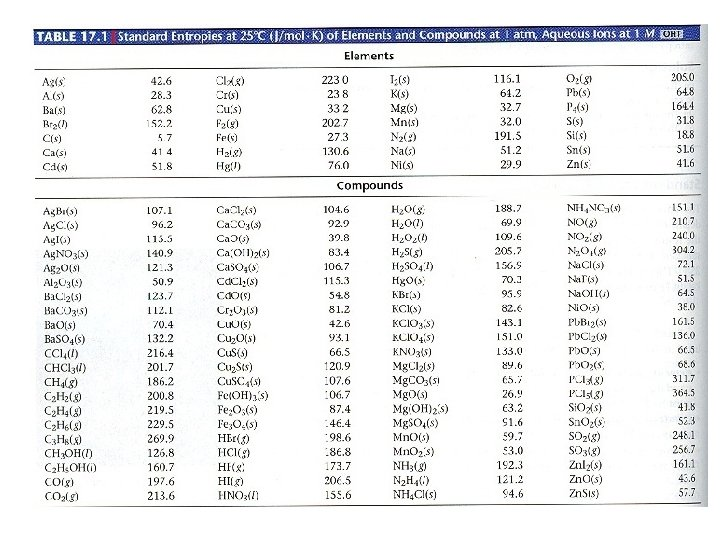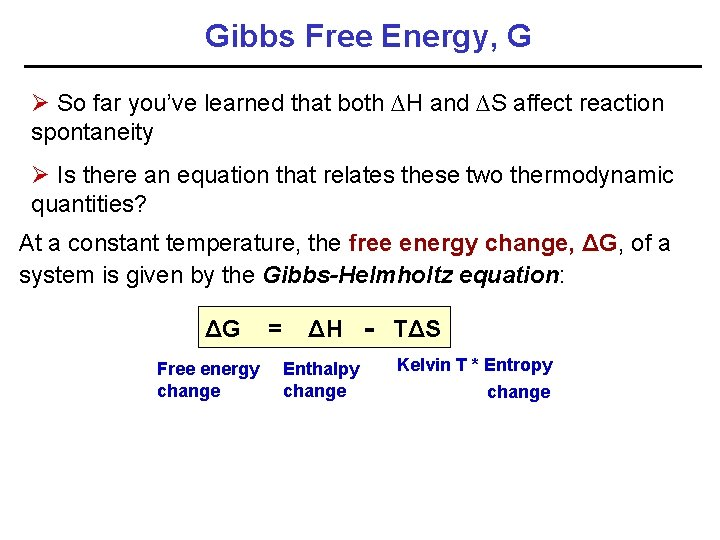Gibbs Free Energy, G Ø So far you’ve learned that both ∆H and ∆S affect reaction spontaneity Ø Is there an equation that relates these two thermodynamic quantities? At a constant temperature, the free energy change, ΔG, of a system is given by the Gibbs-Helmholtz equation: ΔG = ΔH - TΔS Free energy change Enthalpy change Kelvin T * Entropy change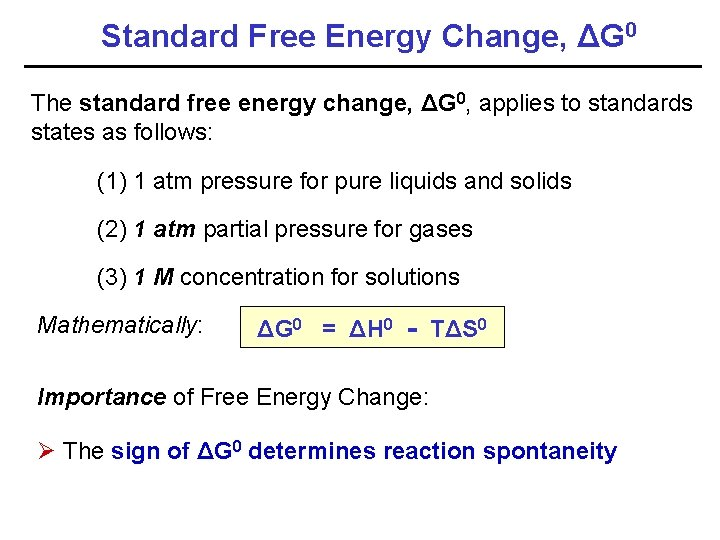Standard Free Energy Change, ΔG 0 The standard free energy change, ΔG 0, applies to standards states as follows: (1) 1 atm pressure for pure liquids and solids (2) 1 atm partial pressure for gases (3) 1 M concentration for solutions Mathematically: ΔG 0 = ΔH 0 - TΔS 0 Importance of Free Energy Change: Ø The sign of ΔG 0 determines reaction spontaneity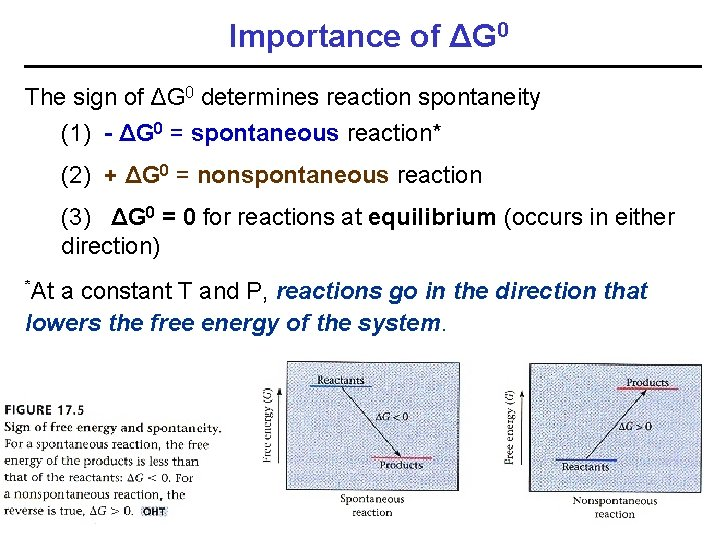Importance of ΔG 0 The sign of ΔG 0 determines reaction spontaneity (1) - ΔG 0 = spontaneous reaction* (2) + ΔG 0 = nonspontaneous reaction (3) ΔG 0 = 0 for reactions at equilibrium (occurs in either direction) *At a constant T and P, reactions go in the direction that lowers the free energy of the system.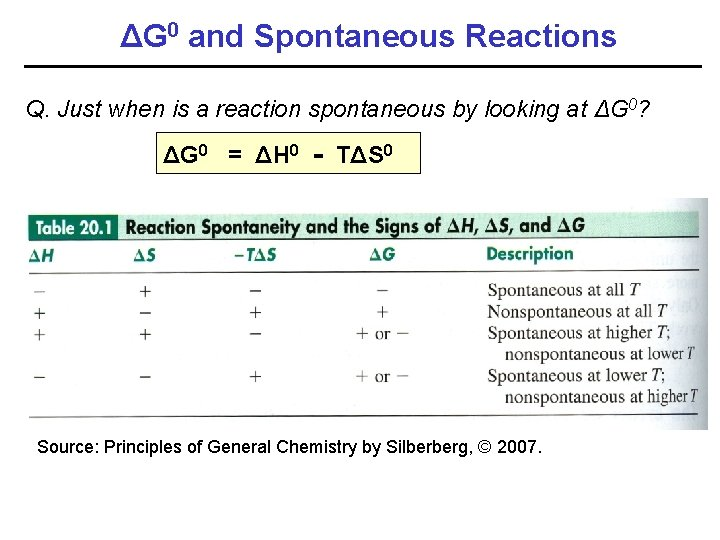ΔG 0 and Spontaneous Reactions Q. Just when is a reaction spontaneous by looking at ΔG 0? ΔG 0 = ΔH 0 - TΔS 0 Source: Principles of General Chemistry by Silberberg, © 2007.ΔG 0 versus ΔG ΔG 0 vs. ΔG 0 = free energy of the system at standard conditions [Pgas = 1 atm; [ ] = 1 M for species in solution (aq)] v Fixed during a reaction under std. conditions ΔG = free energy of the system at any given condition [i. e. at any point in the reaction] v Changes during a reactionImportance of ΔG 0 For a given chemical reaction a. A + b. B → c. C + d. D the free energy of the reaction is given by the equation ∆G = ∆G 0 + RT ln Q Reaction quotient Std. free energy Gas constant Kelvin temp. Q = [C]c [D]d [A]a [B]b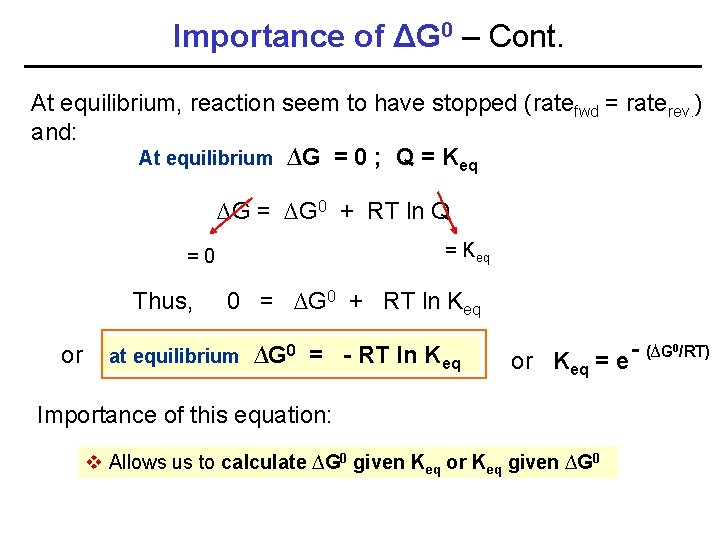Importance of ΔG 0 – Cont. At equilibrium, reaction seem to have stopped (ratefwd = raterev. ) and: At equilibrium ∆G = 0 ; Q = Keq ∆G = ∆G 0 + RT ln Q = Keq =0 Thus, or 0 = ∆G 0 + RT ln Keq at equilibrium ∆G 0 = - RT ln Keq - (∆G 0/RT) or Keq = e Importance of this equation: v Allows us to calculate ∆G 0 given Keq or Keq given ∆G 0